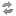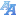Exam dates(version: 188)
Browse before 2020/2021 winter

Type: all courses ---Environment CentreCentre for Theoretical Study;Institute of the History of Charles University and Archive of Charles UniversityRectorateCatholic Theological FacultyProtestant Theological FacultyHussite Theological FacultyFaculty of LawFirst Faculty of MedicineSecond Faculty of MedicineThird Faculty of MedicineFaculty of Medicine in PilsenFaculty of Medicine in Hradec KrálovéFaculty of Pharmacy in Hradec KrálovéFaculty of ArtsFaculty of ScienceFaculty of Mathematics and PhysicsFaculty of EducationFaculty of Social SciencesFaculty of Physical Education and SportFaculty of HumanitiesInstitute for Language and Preparatory StudiesCentral Library of Charles UniversityCentre for Economic Research and Graduate EducationCentre for Knowledge and Technology Transfer---Astronomical Institute of Charles University (32-AUUK)Blíže nespecifikované praxe (32-#PRX)Computer Science Institute of Charles University (32-IUUK)Department of Algebra (32-KA)Department of Applied Mathematics (32-KAM)Department of Atmospheric Physics (32-KFA)Department of Condensed Matter Physics (32-KFKL)Department of Distributed and Dependable Systems (32-KDSS)Department of Geophysics (32-KG)Department of Chemical Physics and Optics (32-KCHFO)Department of Language Education (32-KJP)Department of Low Temperature Physics (32-KFNT)Department of Macromolecular Physics (32-KMF)Department of Mathematical Analysis (32-KMA)Department of Mathematics Education (32-KDM)Department of Numerical Mathematics (32-KNM)Department of Physical Education (32-KTV)Department of Physics Education (32-KDF)Department of Physics of Materials (32-KFM)Department of Probability and Mathematical Statistics (32-KPMS)Department of Software and Computer Science Education (32-KSVI)Department of Software Engineering (32-KSI)Department of Surface and Plasma Science (32-KFPP)Department of Theoretical Computer Science and Mathematical Logic (32-KTIML)Institute of Formal and Applied Linguistics (32-UFAL)Institute of Particle and Nuclear Physics (32-UCJF)Institute of Physics of Charles University (32-FUUK)Institute of Theoretical Physics (32-UTF)Laboratory of General Physics Education (32-KVOF)Matematický ústav AV ČR, v.v.i. (32-MUAV)Mathematical Institute of Charles University (32-MUUK)Network and Labs Management Center (32-SISAL)Student Affairs Department (32-STUD)---Numerical Mathematics - NMAI042Mathematical problems of non-mathematicians - NMAT100Seminář z mechaniky kontinua - NMMO461Saddle Point Problems and Their Solution - NMMO537Fundamentals of Numerical Mathematics - NMNM201Introduction to Numerical Mathematics - NMNM211Analysis of Matrix Calculations 1 - NMNM331Analysis of Matrix Calculations 2 - NMNM332Introduction to Mathematical Modelling - NMNM334Introduction to the Finite Element Method - NMNM336Analysis of Matrix Calculations 1 (M) - NMNM931Fractals and Chaotic Dynamics - NMNV361Functional Analysis - NMNV401Numerical Software 1 - NMNV403Numerical Software 2 - NMNV404Finite Element Method 1 - NMNV405Nonlinear differential equations - NMNV406Algorithms for matrix iterative methods - NMNV411Analysis of matrix iterative methods - principles and interconnections - NMNV412Finite Element Method 2 - NMNV436Seminar in Numerical Mathematics - NMNV451Techniques for a posteriori error estimation - NMNV461Numerical Modelling of Electrical Engineering Problems - NMNV462Modelling of materials - theory, model reduction and efficient numerical methods - NMNV463Numerical Linear Algebra for data science and informatics - NMNV468Solution of Nonlinear Algebraic Equations - NMNV501Inverse Problems and Regularization - NMNV531Parallel Matrix Computations - NMNV532Sparse Matrices in Numerical Mathematics - NMNV533Numerical Optimization Methods - NMNV534Numerical Solution of Evolutionary Equations - NMNV536Mathematical Methods in Fluid Mechanics 1 - NMNV537Mathematical Methods in Fluid Mechanics 2 - NMNV538Numerical Solution of ODE - NMNV539Fundamentals of Discontinuous Galerkin Method - NMNV540Shape and Material Optimisation 1 - NMNV541Shape and Material Optimisation 2 - NMNV542Approximation of functions 1 - NMNV543Bifurcation Analysis of Dynamical Systems 1 - NMNV561Bifurcation Analysis of Dynamical Systems 2 - NMNV562High-Performance Computing for Computational Science - NMNV565Approximation of functions 2 - NMNV568Contemporary Problems in Numerical Mathematics - NMNV623--- only future only the free ones 20501002505001000
* no data found

Charles University | Information system of Charles University | http://www.cuni.cz/UKEN-329.html